Question

# Write a program that receives a positive integer n from the user and then calculates the...

Write a program that receives a positive integer n from the user and then calculates the sum below by using a loop. Your program needs to ask the user to re-enter a different number if the user enters a non-positive one. Display the result. 1 SUM 1 2 3 ... n x x n = = = + + + +

Code:

#include<iostream>
using namespace std;
int main()
{
int n,i,sum=0;
cout<<"Enter a number to find the sum of numbers below it:";
cin>>n;/*Reading number from the user*/
while(n<=0)
{/*This loop execute until user enter a positive number*/
cout<<"Number should be positive"<<endl;
cout<<"Enter a number to find the sum of numbers below it:";
cin>>n;
}
for(i=1;i<=n;i++)
{/*This loop iterates for 1 to n*/
sum+=i;
/*Here we calculate the sum*/
}
cout<<"Sum is: "<<sum;
/*Finall we print the sum*/
}

Output: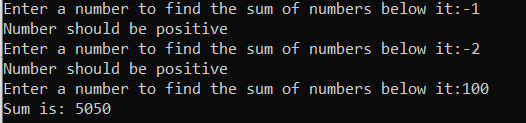indentation: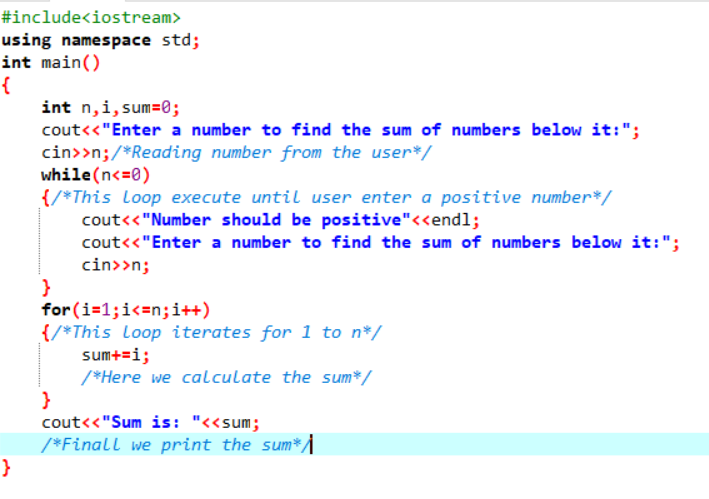#### Earn Coins

Coins can be redeemed for fabulous gifts.

Similar Homework Help Questions
• ### 1. (sumFrom1.cpp) Write a program that will ask the user for a positive integer value. The...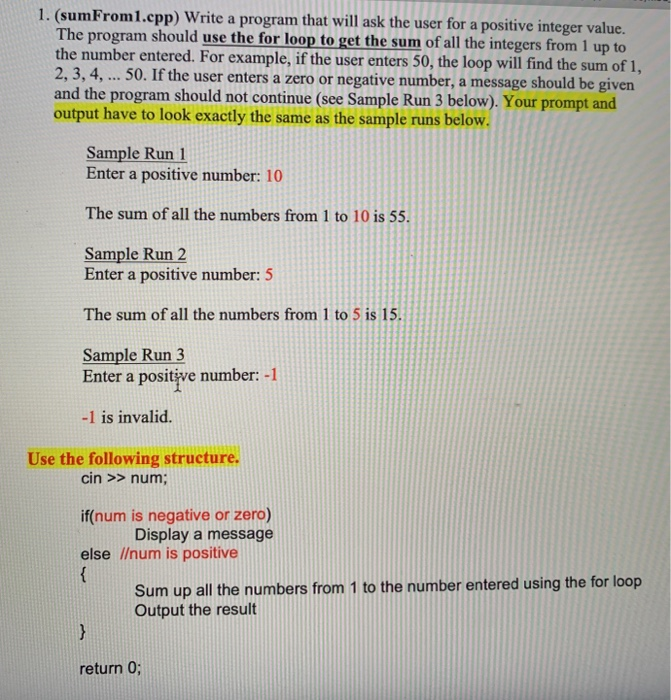1. (sumFrom1.cpp) Write a program that will ask the user for a positive integer value. The program should use the for loop to get the sum of all the integers from 1 up to the number entered. For example, if the user enters 50, the loop will find the sum of 1, 2, 3, 4, ... 50. If the user enters a zero or negative number, a message should be given and the program should not continue (see Sample Run...

• ### In Python, write a program that asks the user for a positive integer number. If the...

In Python, write a program that asks the user for a positive integer number. If the user enters anything else, the program would ask the user to re-enter a number or enter -1 to quit. If it is a positive number, the program then prints out a multiplication table that goes up to that number. For example, if the user enters 10, the output would look something like this. https://www.vectorstock.com/royalty-free-vector/multiplication-table-on-white-background-vector-2721686

• ### In java, write a program that gets 10 integer numbers from the user using user input,...

In java, write a program that gets 10 integer numbers from the user using user input, and then calculates and display the sum of the numbers that have been read.   Program Requirements: Write the program in three versions with three loops. Put all three loops in the main method of your source code. version1:  use a while loop. version2:  use a do-while loop. version 3:  use a for loop. For each version, use a loop to input 10 int numbers from the user...

• ### C++ only Implement a program that prompts a user to enter a number N where N...

C++ only Implement a program that prompts a user to enter a number N where N is a positive number. The program must validate a user input and ask a user to re-enter until an input is valid. Implement a function that pass N as an argument and return a result of calculates N! (N-factorial): The function must use loop for the implementation. Hint: Factorial: N! = N * (N-1) * (N-2) * …. * 1 ( This is only...

• ### C++ coding answer 5. Write a program that asks the user for a positive integer value....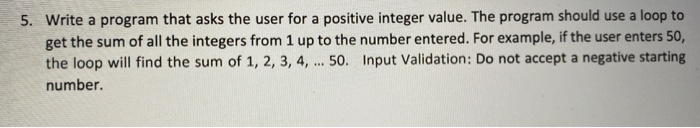C++ coding answer 5. Write a program that asks the user for a positive integer value. The program should use a loop to get the sum of all the integers from 1 up to the number entered. For example, if the user enters 50, the loop will find the sum of 1, 2, 3, 4, ... 50. Input Validation: Do not accept a negative starting number.

• ### Student ID: 123 Write a C+ program with the following specifications: a. Define a C++ function (name it function_Student...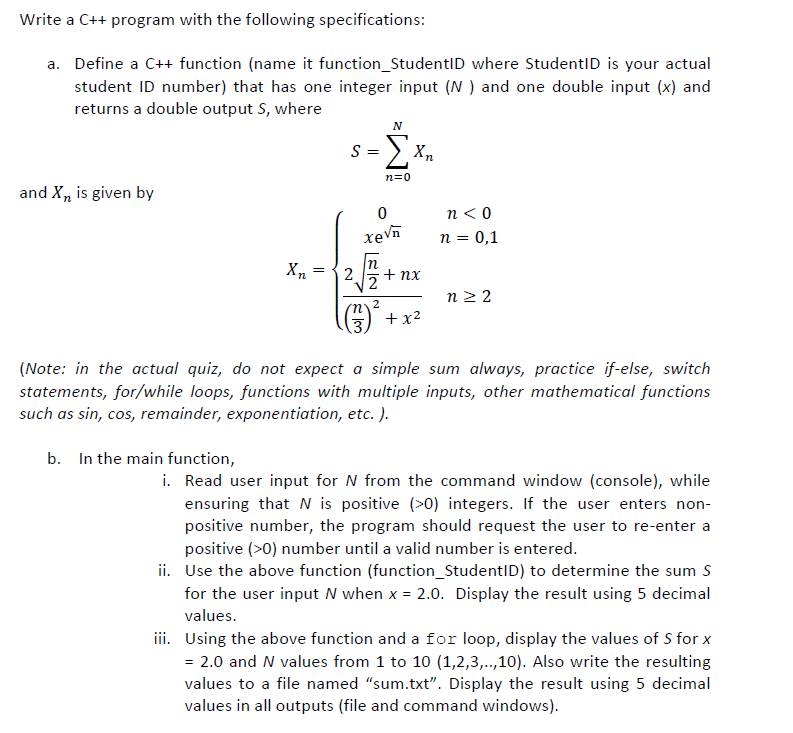Student ID: 123 Write a C+ program with the following specifications: a. Define a C++ function (name it function_StudentlD where StudentID is your actual student ID number) that has one integer input (N) and one double input (x) and returns a double output S, where N S = n 0 and X2 is given by 0 xeVn n 0,1 Хл —{2. nx 2 n 2 2 m2 x2 3 (Note: in the actual quiz, do not expect a always, practice...

• ### Write a program that calculates the average of a stream of non-negative numbers. The user can...

Write a program that calculates the average of a stream of non-negative numbers. The user can enter as many non-negative numbers as they want, and they will indicate that they are finished by entering a negative number. For this program, zero counts as a number that goes into the average. Of course, the negative number should not be part of the average (and, for this program, the average of 0 numbers is 0). You must use a method to read...

• ### Summary: Write a C program that prompts the user to enter 2 positive integer numbers, display...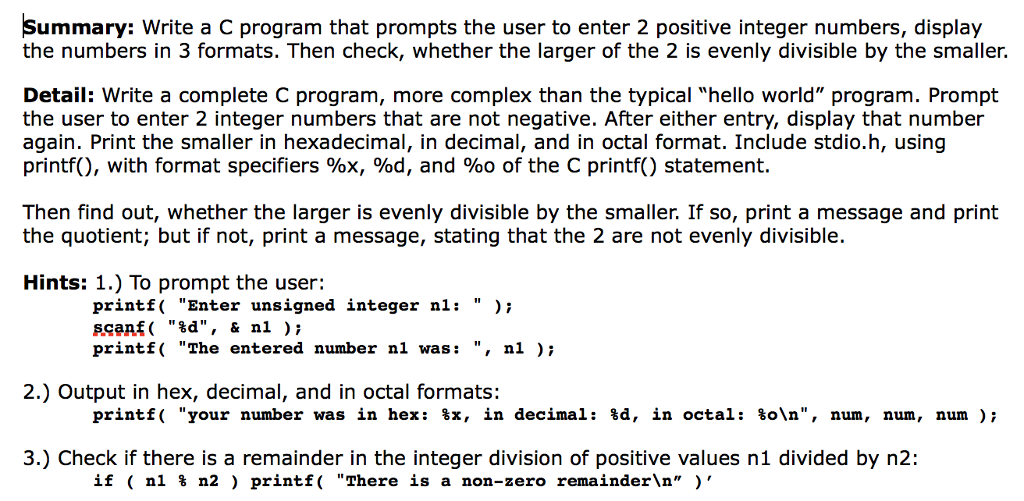Summary: Write a C program that prompts the user to enter 2 positive integer numbers, display the numbers in 3 formats. Then check, whether the larger of the 2 is evenly divisible by the smaller. Detail: Write a complete C program, more complex than the typical "hello world" program. Prompt the user to enter 2 integer numbers that are not negative. After either entry, display that number again. Print the smaller in hexadecimal, in decimal, and in octal format. Include...

• ### use C++ to write the following program. needs to ask the user for n The user...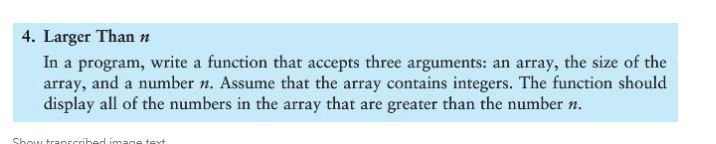use C++ to write the following program. needs to ask the user for n The user must put in those 5 values, probably using a for loop. NOTE: You can assume the number of values to be entered is 5. My attempted program below: (not correct, but just a basis) 4. Larger Than n In a program, write a function that accepts three arguments: an array, the size of the array, and a number n. Assume that the array contains...

• ### Write a complete C++ program that do following. 1) Read a positive integer from the user...

Write a complete C++ program that do following. 1) Read a positive integer from the user with proper prompt. Assume that this user is nice and he/she would not start the integer with digit 0. 2) Create a size 10 array as counters for digits 0 - 9. 3) Use a while loop for processing a) Single out the current last digit using modular 10 technique b) Increment the corresponding counter through the index derived in a). 4) At the...